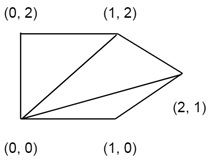# Minimum Cost Polygon Triangulation

When nonintersecting diagonals are forming a triangle in a polygon, it is called the triangulation. Our task is to find a minimum cost of triangulation.

The cost of triangulation is the sum of the weights of its component triangles. We can find the weight of each triangle by adding their sides, in other words, the weight is the perimeter of the triangle.

## Input and Output

Input:
The points of a polygon. {(0, 0), (1, 0), (2, 1), (1, 2), (0, 2)}Output:
The total cost of the triangulation. Here the cost of the triangulation is 15.3006.

## Algorithm

minCost(polygon, n)

Here cost() will be used to calculate the perimeter of a triangle.

Input: A set of points to make a polygon, and a number of points.

Output − Minimum cost for triangulation of a polygon.

Begin
if n < 3, then
return 0
define table or order n x n
i := 0

for gap := 0 to n-1, do
for j := gap to n-1, do
if j < i+2, then
table[i,j] := 0
else
table[i, j] = ∞
for k := i+1 to j-1, do
val := table[i, k] + table[k, j] + cost(i, j, k)
if table[i, j] > val
table[i, j] := val
i := i + 1
done
done
return table[0, n-1]
End

## Example

#include <iostream>
#include <cmath>
#include <iomanip>
#define MAX 1000000.0
using namespace std;

struct Point {
int x, y;
};

double min(double x, double y) {
return (x <= y)? x : y;
}

double dist(Point p1, Point p2) {    //find distance from p1 to p2
return sqrt(pow((p1.x-p2.x),2) + pow((p1.y-p2.y),2));
}

double cost(Point triangle[], int i, int j, int k) {
Point p1 = triangle[i], p2 = triangle[j], p3 = triangle[k];
return dist(p1, p2) + dist(p2, p3) + dist(p3, p1);    //the perimeter of the triangle
}

double minimumCost(Point polygon[], int n) {
if (n < 3)    //when polygon has less than 3 points
return 0;
double table[n][n];

for (int gap = 0; gap < n; gap++) {
for (int i = 0, j = gap; j < n; i++, j++) {
if (j < i+2)
table[i][j] = 0.0;
else {
table[i][j] = MAX;

for (int k = i+1; k < j; k++) {
double val = table[i][k] + table[k][j] + cost(polygon,i,j,k);
if (table[i][j] > val)
table[i][j] = val;    //update table data to minimum value
}
}
}
}
return  table[n-1];
}

int main() {
Point points[] = {{0, 0}, {1, 0}, {2, 1}, {1, 2}, {0, 2}};
int n = 5;
cout <<"The minimumcost: " <<minimumCost(points, n);
}

## Output

The minimumcost: 15.3006